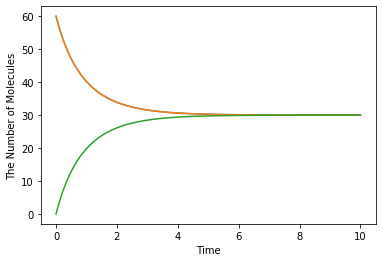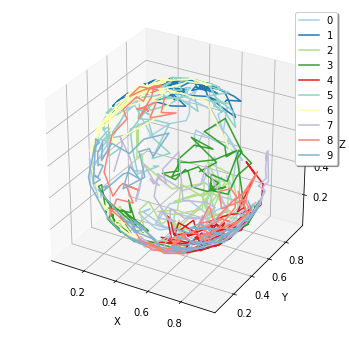# Welcome to E-Cell4 in Jupyter Notebook!¶

## Quick Start¶

### Binding and unbinding reactions¶

Here is an extremely simple example with a reversible binding reaction:

In :
%matplotlib inline
from ecell4.prelude import *

with reaction_rules():
A + B == C | (0.01, 0.3)

run_simulation(10, {'A': 60, 'B': 60})### Diffusion on a spherical surface¶

In :
%matplotlib inline
from ecell4.prelude import *

with species_attributes():
M | {'dimension': 2}
A | {'D': 1.0, 'location': 'M'}

surface = Sphere(ones() * 0.5, 0.49).surface()
obs = FixedIntervalTrajectoryObserver(4e-3)
run_simulation(
0.4, y0={'A': 10}, structures={'M': surface},
solver='spatiocyte', observers=obs)

show(obs)### Plotly¶

Interactive visualizations by Plotly are also available. This part might not works on Google Colab.

In :
%matplotlib inline
from ecell4.prelude import *

In :
with reaction_rules():
A + B == C | (0.01, 0.3)

ret = run_simulation(10, {'A': 60, 'B': 60})

In :
ret.plot(backend='plotly')